# Usage of the sampling_strategy parameter for the different algorithms¶

This example shows the different usage of the parameter sampling_strategy for the different family of samplers (i.e. over-sampling, under-sampling. or cleaning methods).

# Authors: Guillaume Lemaitre <g.lemaitre58@gmail.com>

from collections import Counter

import numpy as np
import matplotlib.pyplot as plt

from imblearn.datasets import make_imbalance

from imblearn.over_sampling import RandomOverSampler
from imblearn.under_sampling import RandomUnderSampler

print(__doc__)

def plot_pie(y):
target_stats = Counter(y)
labels = list(target_stats.keys())
sizes = list(target_stats.values())
explode = tuple([0.1] * len(target_stats))

def make_autopct(values):
def my_autopct(pct):
total = sum(values)
val = int(round(pct * total / 100.0))
return '{p:.2f}%  ({v:d})'.format(p=pct, v=val)
return my_autopct

fig, ax = plt.subplots()
autopct=make_autopct(sizes))
ax.axis('equal')


First, we will create an imbalanced data set from a the iris data set.

iris = load_iris()

print('Information of the original iris data set: \n {}'.format(
Counter(iris.target)))
plot_pie(iris.target)

sampling_strategy = {0: 10, 1: 20, 2: 47}
X, y = make_imbalance(iris.data, iris.target,
sampling_strategy=sampling_strategy)

print('Information of the iris data set after making it'
' imbalanced using a dict: \n sampling_strategy={} \n y: {}'
.format(sampling_strategy, Counter(y)))
plot_pie(y)

•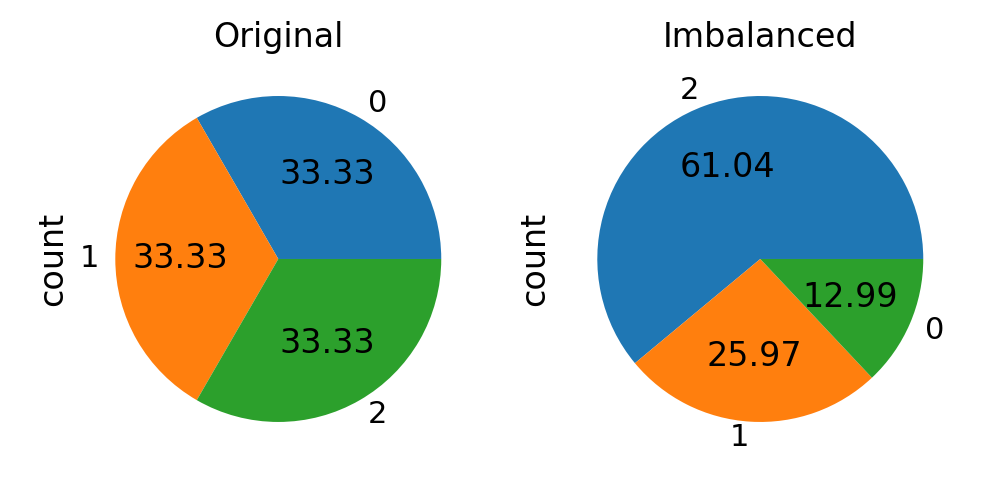•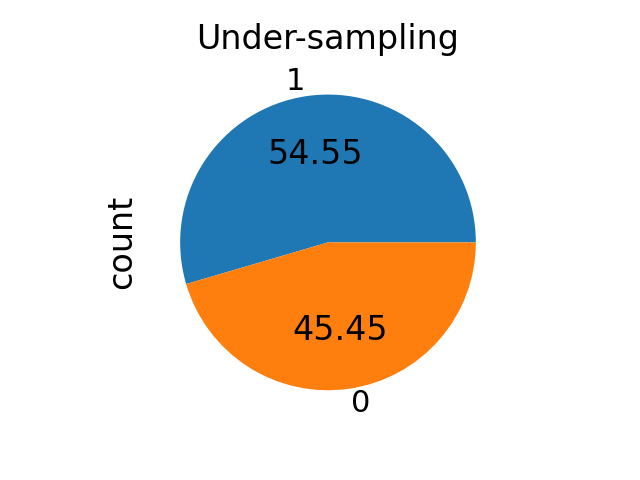Out:

Information of the original iris data set:
Counter({0: 50, 1: 50, 2: 50})
Information of the iris data set after making it imbalanced using a dict:
sampling_strategy={0: 10, 1: 20, 2: 47}
y: Counter({2: 47, 1: 20, 0: 10})


## Using sampling_strategy in resampling algorithms¶

### sampling_strategy as a float¶

sampling_strategy can be given a float. For under-sampling methods, it corresponds to the ratiodefined bywhereandare the number of samples in the majority class after resampling and the number of samples in the minority class, respectively.

# select only 2 classes since the ratio make sense in this case
binary_mask = np.bitwise_or(y == 0, y == 2)

sampling_strategy = 0.8

rus = RandomUnderSampler(sampling_strategy=sampling_strategy)
X_res, y_res = rus.fit_resample(binary_X, binary_y)
print('Information of the iris data set after making it '
'balanced using a float and an under-sampling method: \n '
'sampling_strategy={} \n y: {}'
.format(sampling_strategy, Counter(y_res)))
plot_pie(y_res)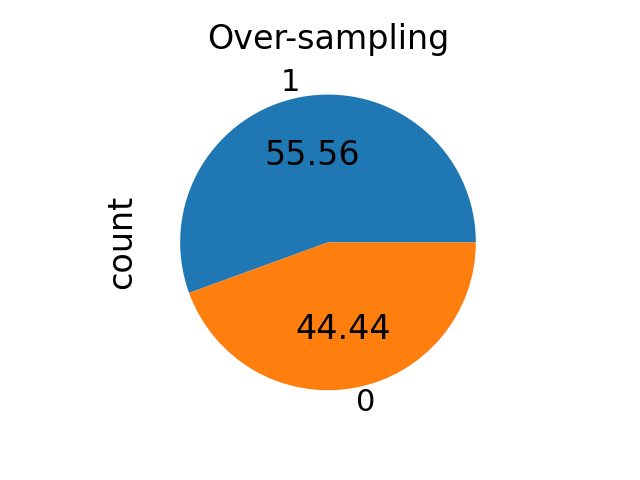Out:

Information of the iris data set after making it balanced using a float and an under-sampling method:
sampling_strategy=0.8
y: Counter({2: 12, 0: 10})


For over-sampling methods, it correspond to the ratiodefined bywhereandare the number of samples in the minority class after resampling and the number of samples in the majority class, respectively.

ros = RandomOverSampler(sampling_strategy=sampling_strategy)
X_res, y_res = ros.fit_resample(binary_X, binary_y)
print('Information of the iris data set after making it '
'balanced using a float and an over-sampling method: \n '
'sampling_strategy={} \n y: {}'
.format(sampling_strategy, Counter(y_res)))
plot_pie(y_res)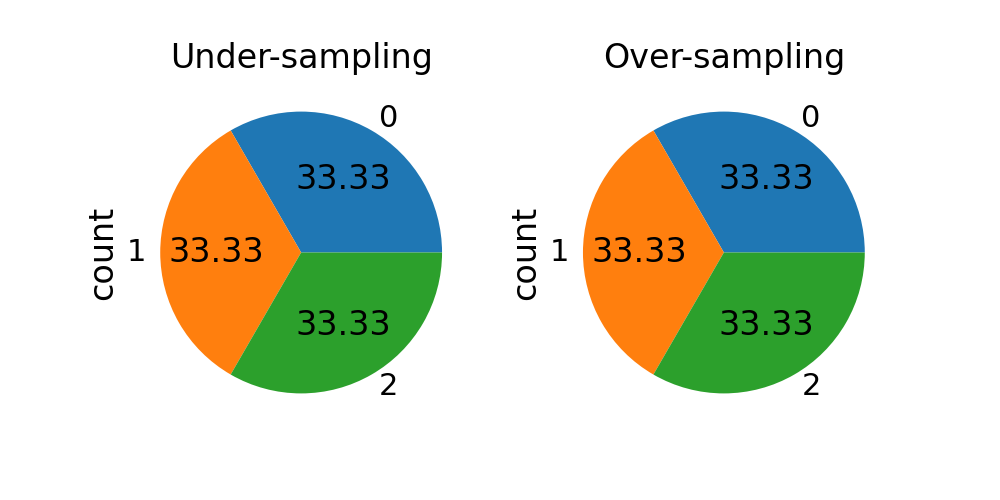Out:

Information of the iris data set after making it balanced using a float and an over-sampling method:
sampling_strategy=0.8
y: Counter({2: 47, 0: 37})


### sampling_strategy has a str¶

sampling_strategy can be given as a string which specify the class targeted by the resampling. With under- and over-sampling, the number of samples will be equalized.

Note that we are using multiple classes from now on.

sampling_strategy = 'not minority'

rus = RandomUnderSampler(sampling_strategy=sampling_strategy)
X_res, y_res = rus.fit_resample(X, y)
print('Information of the iris data set after making it '
'balanced by under-sampling: \n sampling_strategy={} \n y: {}'
.format(sampling_strategy, Counter(y_res)))
plot_pie(y_res)

sampling_strategy = 'not majority'

ros = RandomOverSampler(sampling_strategy=sampling_strategy)
X_res, y_res = ros.fit_resample(X, y)
print('Information of the iris data set after making it '
'balanced by over-sampling: \n sampling_strategy={} \n y: {}'
.format(sampling_strategy, Counter(y_res)))
plot_pie(y_res)

•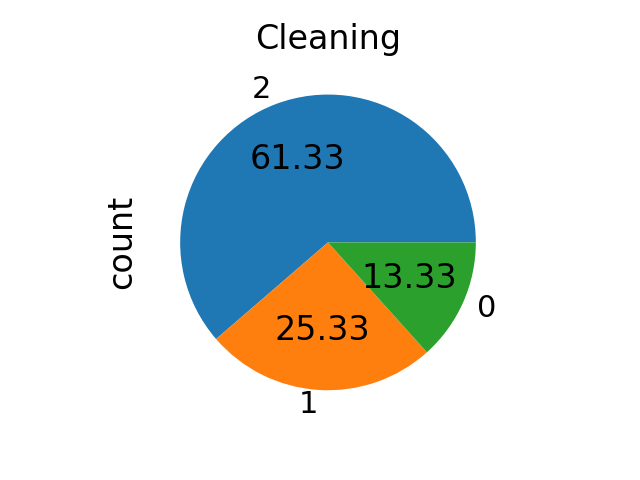•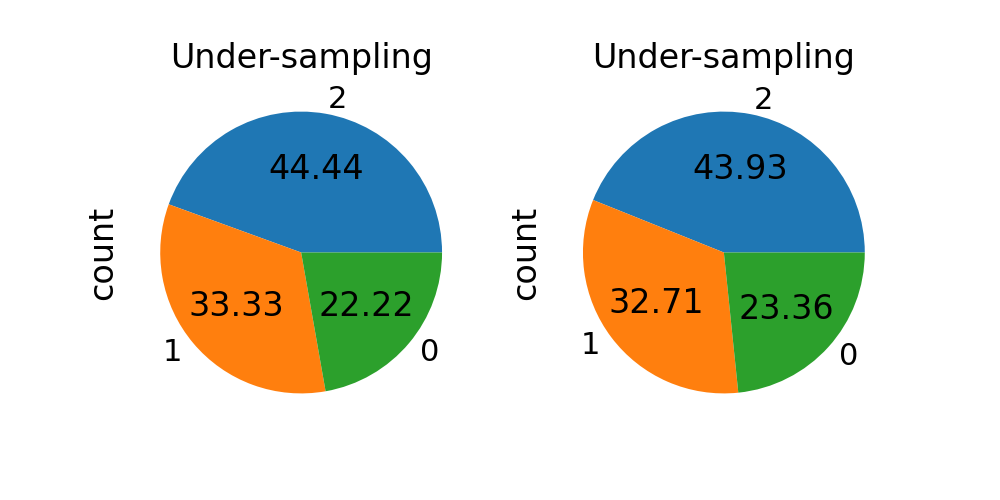Out:

Information of the iris data set after making it balanced by under-sampling:
sampling_strategy=not minority
y: Counter({0: 10, 1: 10, 2: 10})
Information of the iris data set after making it balanced by over-sampling:
sampling_strategy=not majority
y: Counter({0: 47, 1: 47, 2: 47})


With cleaning method, the number of samples in each class will not be equalized even if targeted.

sampling_strategy = 'not minority'
X_res, y_res = tl.fit_resample(X, y)
print('Information of the iris data set after making it '
'balanced by cleaning sampling: \n sampling_strategy={} \n y: {}'
.format(sampling_strategy, Counter(y_res)))
plot_pie(y_res)Out:

/home/circleci/project/imblearn/utils/_validation.py:638: FutureWarning: Pass sampling_strategy=not minority as keyword args. From version 0.9 passing these as positional arguments will result in an error
FutureWarning)
Information of the iris data set after making it balanced by cleaning sampling:
sampling_strategy=not minority
y: Counter({2: 47, 1: 20, 0: 10})


### sampling_strategy as a dict¶

When sampling_strategy is a dict, the keys correspond to the targeted classes. The values correspond to the desired number of samples for each targeted class. This is working for both under- and over-sampling algorithms but not for the cleaning algorithms. Use a list instead.

sampling_strategy = {0: 10, 1: 15, 2: 20}

rus = RandomUnderSampler(sampling_strategy=sampling_strategy)
X_res, y_res = rus.fit_resample(X, y)
print('Information of the iris data set after making it '
'balanced by under-sampling: \n sampling_strategy={} \n y: {}'
.format(sampling_strategy, Counter(y_res)))
plot_pie(y_res)

sampling_strategy = {0: 25, 1: 35, 2: 47}

ros = RandomOverSampler(sampling_strategy=sampling_strategy)
X_res, y_res = ros.fit_resample(X, y)
print('Information of the iris data set after making it '
'balanced by over-sampling: \n sampling_strategy={} \n y: {}'
.format(sampling_strategy, Counter(y_res)))
plot_pie(y_res)

•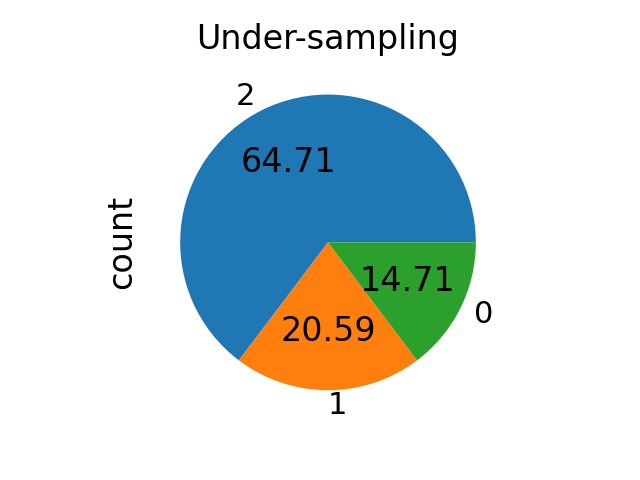•Out:

Information of the iris data set after making it balanced by under-sampling:
sampling_strategy={0: 10, 1: 15, 2: 20}
y: Counter({2: 20, 1: 15, 0: 10})
Information of the iris data set after making it balanced by over-sampling:
sampling_strategy={0: 25, 1: 35, 2: 47}
y: Counter({2: 47, 1: 35, 0: 25})


### sampling_strategy as a list¶

When sampling_strategy is a list, the list contains the targeted classes. It is used only for cleaning methods and raise an error otherwise.

sampling_strategy = [0, 1, 2]
X_res, y_res = tl.fit_resample(X, y)
print('Information of the iris data set after making it '
'balanced by cleaning sampling: \n sampling_strategy={} \n y: {}'
.format(sampling_strategy, Counter(y_res)))
plot_pie(y_res)Out:

Information of the iris data set after making it balanced by cleaning sampling:
sampling_strategy=[0, 1, 2]
y: Counter({2: 47, 1: 20, 0: 10})


### sampling_strategy as a callable¶

When callable, function taking y and returns a dict. The keys correspond to the targeted classes. The values correspond to the desired number of samples for each class.

def ratio_multiplier(y):
multiplier = {1: 0.7, 2: 0.95}
target_stats = Counter(y)
for key, value in target_stats.items():
if key in multiplier:
target_stats[key] = int(value * multiplier[key])
return target_stats

X_res, y_res = (RandomUnderSampler(sampling_strategy=ratio_multiplier)
.fit_resample(X, y))

print('Information of the iris data set after balancing using a callable'
' mode:\n ratio={} \n y: {}'.format(ratio_multiplier, Counter(y_res)))
plot_pie(y_res)

plt.show()Out:

Information of the iris data set after balancing using a callable mode:
ratio=<function ratio_multiplier at 0x7f45342bff80>
y: Counter({2: 44, 1: 14, 0: 10})


Total running time of the script: ( 0 minutes 4.392 seconds)

Estimated memory usage: 12 MB

Gallery generated by Sphinx-Gallery